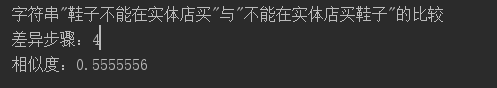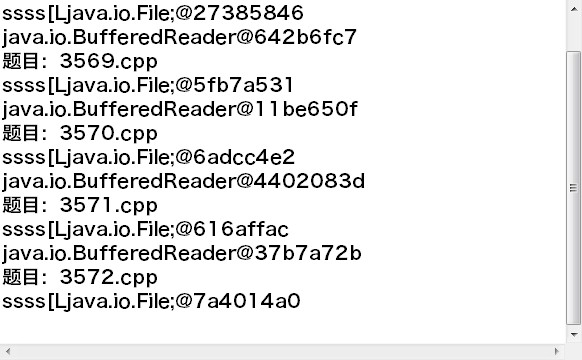• 相似度匹配算法实际是计算字符串之间的编辑距离。 经常刷算法的同学，就应该知道这个是一个可迭代可递归实现的中难度的leetcode算法。 详细思路可以在网上找找，我就直接给出对应的的代码！ 二维dp算法 /** *...

相似度匹配算法实际是计算字符串之间的编辑距离。

经常刷算法的同学，就应该知道这个是一个可迭代可递归实现的中难度的leetcode算法。

详细思路可以在【思路分析指引】看看，我就直接给出对应的的代码！

二维dp算法

/**
* 相似du匹配算法，约靠近返回的值越高
* @param target
* @param findOb
* @return
*/
public static double matchStr(String target ,Object findOb ) {
//length
String find = String.valueOf(findOb);
int Length1 = target.length();
int Length2 = find.length();
int distance = 0;
if (Length1 == 0) {
distance = Length2;
}
if (Length2 == 0) {
distance = Length1;
}
if (Length1 != 0 && Length2 != 0) {
int[][] dp = new int[Length1 + 1][Length2 + 1];
//编号
int initValue = 0;
for (int i = 0; i <= Length1; i++) {
dp[i] = initValue;
initValue++;
}
initValue = 0;
for (int i = 0; i <= Length2; i++) {
dp[i] = initValue;
initValue++;
}
char[] targetArr = target.toCharArray();
char[] findArr = find.toCharArray();
for (int i = 1; i <= Length1; i++) {
for (int j = 1; j <= Length2; j++) {
if (targetArr[i - 1] == findArr[j - 1]) {
distance = 0;
} else {
distance = 1;
}
dp[i][j] = Math.min(
dp[i - 1][j] + 1,  //  删除
dp[i][j - 1] + 1,       // 插入
dp[i - 1][j - 1] + 1     // 替换
)
}
}
distance = dp[Length1][Length2];
}
double v = 1 - 1.0 * distance / (Length1 > Length2 ? Length1 : Length2);
return v;
}

上面的代码还可以进行状态压缩优化，我们还需要一个额外的变量 pre 来时刻保存 (i-1,j-1) 的值。推导公式就可以从二维的：

dp[i][j] = min(dp[i-1][j] , dp[i-1][j-1] , dp[i][j-1]) + 1

转化为一维的：

dp[i] = min(dp[i-1], pre, dp[i]) + 1

一维dp算法

public static int matchStr(String target ,Object findOb ) {
//length
Double v = 0d;
String find = String.valueOf(findOb).toLowerCase();
int targetLength = target.length();
int findLength = find.length();
int distance = 0;
if (targetLength != 0 && findLength != 0) {
target=target.toLowerCase();
if(find.contains(target)){
v = Double.valueOf(1000-findLength+targetLength);
}else{
int max = Math.max(targetLength, findLength);
int[] dp = new int[max+1];
//编号
for (int i = 0; i <= max; i++) {
dp[i] = i;
}
char[] targetArr = target.toCharArray();
char[] findArr = find.toCharArray();
for (int i = 1; i <= targetLength; i++) {
int temp = dp;
for (int j = 1; j <= findLength; j++) {
int pre = temp;
temp = dp[j];
if (targetArr[i - 1] == findArr[j - 1]) {
dp[j] = pre;
} else {
dp[j] = Math.min(Math.min(dp[j - 1], pre), dp[j]) + 1;
}
}
}
distance = dp[findLength];
v = (1 - 1.0 * distance / (targetLength > findLength ? targetLength : findLength)) * 1000;
}
}
return v.intValue();
}

展开全文• 中文文本相似度匹配算法 simHash 海明距离 IK分词 完整的可运行的示例代码 包含simHash 算法，使用IK 对中文文本进行分词处理
• 使用JAVA编写的，分词、TFIDF相似度计算，K临近法聚类
• Java实现相似度匹配算法

千次阅读 2018-12-09 17:03:00
* 相似度匹配算法 * （据说）由俄国人Vladimir Levenshtein在1965年发明 * 原理：返回将第一个字符串转换(删除、插入、替换)成第二个字符串的编辑次数。 * 次数越少，意味着字符串相似度越高 */ public ...
/**
* 相似度匹配算法
* 原理：返回将第一个字符串转换(删除、插入、替换)成第二个字符串的编辑次数。
* 次数越少，意味着字符串相似度越高
*/

public class Test {

public static void main(String[] args) {

String Str_1="abcdefghi5klmuvwxyz";
String Str_2="akcdefghijkl2uvwxz";
//length
int Length1=Str_1.length();
int Length2=Str_2.length();

int Distance=0;
if (Length1==0) {
Distance=Length2;
}
if(Length2==0)
{
Distance=Length1;
}
if(Length1!=0&&Length2!=0){
int[][] Distance_Matrix=new int[Length1+1][Length2+1];
//编号
int Bianhao=0;
for (int i = 0; i <= Length1; i++) {
Distance_Matrix[i]=Bianhao;
Bianhao++;
}
Bianhao=0;
for (int i = 0; i <=Length2; i++) {
Distance_Matrix[i]=Bianhao;
Bianhao++;
}

char[] Str_1_CharArray=Str_1.toCharArray();
char[] Str_2_CharArray=Str_2.toCharArray();

for (int i = 1; i <= Length1; i++) {
for(int j=1;j<=Length2;j++){
if(Str_1_CharArray[i-1]==Str_2_CharArray[j-1]){
Distance=0;
}
else{
Distance=1;
}

int Temp1=Distance_Matrix[i-1][j]+1;
int Temp2=Distance_Matrix[i][j-1]+1;
int Temp3=Distance_Matrix[i-1][j-1]+Distance;

Distance_Matrix[i][j]=Temp1>Temp2?Temp2:Temp1;
Distance_Matrix[i][j]=Distance_Matrix[i][j]>Temp3?Temp3:Distance_Matrix[i][j];

}

}

Distance=Distance_Matrix[Length1][Length2];
}

double Aerfa=1-1.0*Distance/(Length1>Length2?Length1:Length2);
System.out.println(Aerfa);
}

}

展开全文java 文本相似度匹配算法
• Java字符串相似度匹配

万次阅读 2018-11-16 00:30:31
System.out.println("相似度："+similarity); } //得到最小值 private static int min(int... is) { int min = Integer.MAX_VALUE; for (int i : is) { if (min > i) { min = i; } } return min; } } ...

package com.xfl.boot.common.utils;

/**
* Created by XFL
* time on 2018/11/16 0:10
* description:
*/
public class SimilarityUtils {
public static void main(String[] args) {
//要比较的两个字符串
String str1 = "鞋子不能在实体店买";
String str2 = "不能在实体店买鞋子";
levenshtein(str1.toLowerCase(),str2.toLowerCase());
}

/**
*
* @param str1
* @param str2
*/
public static void levenshtein(String str1,String str2) {
//计算两个字符串的长度。
int len1 = str1.length();
int len2 = str2.length();
//建立上面说的数组，比字符长度大一个空间
int[][] dif = new int[len1 + 1][len2 + 1];
//赋初值，步骤B。
for (int a = 0; a <= len1; a++) {
dif[a] = a;
}
for (int a = 0; a <= len2; a++) {
dif[a] = a;
}
//计算两个字符是否一样，计算左上的值
int temp;
for (int i = 1; i <= len1; i++) {
for (int j = 1; j <= len2; j++) {
if (str1.charAt(i - 1) == str2.charAt(j - 1)) {
temp = 0;
} else {
temp = 1;
}
//取三个值中最小的
dif[i][j] = min(dif[i - 1][j - 1] + temp, dif[i][j - 1] + 1,
dif[i - 1][j] + 1);
}
}
System.out.println("字符串\""+str1+"\"与\""+str2+"\"的比较");
//取数组右下角的值，同样不同位置代表不同字符串的比较
System.out.println("差异步骤："+dif[len1][len2]);
//计算相似度
float similarity =1 - (float) dif[len1][len2] / Math.max(str1.length(), str2.length());
System.out.println("相似度："+similarity);
}

//得到最小值
private static int min(int... is) {
int min = Integer.MAX_VALUE;
for (int i : is) {
if (min > i) {
min = i;
}
}
return min;
}
}展开全文• 【实例简介】代码相似度算法【实例截图】【核心代码】public String getPreProcessedCode(String filePath) {// TODO Auto-generated method stubString code="";try{BufferedReader br = new BufferedReader (new ...

【实例简介】

代码相似度算法

【实例截图】【核心代码】

public String getPreProcessedCode(String filePath) {

// TODO Auto-generated method stub

String code="";

try{

StringBuffer buf = new StringBuffer();

String line;

buf.append(line "\n");

}

//删除所有注释

int pos1 = 0,pos2 = 0;

int len = code.length();

boolean isString = false;

StringBuffer ret = new StringBuffer();

while(pos1

pos2 ;

if(isString){

if(pos2

if(code.substring(pos2, pos2 1).equals("\"") && !code.subSequence(pos2-1, pos2).equals("\\")){

isString = false;

ret.append(delVariables(code.substring(pos1, pos2 1)));

pos1 = pos2 1;

}

}else{

break;

}

}else{

if(pos2

if(code.substring(pos2, pos2 1).equals("\"")){

isString = true;

ret.append(delVariables(code.substring(pos1, pos2)));

pos1 = pos2;

}

}else{

ret.append(delVariables(code.substring(pos1, code.length())));

break;

}

}

}

code = ret.toString();

//删除所有空格和换行

code=code.replaceAll("\\s", "");

br.close();

}catch(Exception e){

e.printStackTrace();

}

return code;

}

展开全文• java算法之-相似度匹配

千次阅读 2015-10-30 15:30:45
package ... /** * 比较两个字符串的相似度 */ public class SimilarityUtil { private static int LowerOfThree(int first, int second, int third) { int min = Math.min(first, second); ...java 操作 开发
• Java字符串匹配相似度算法

千次阅读 2018-12-19 11:34:50
* 采用动态规划的方法（字符串匹配相似度） * @param source 源 * @param target 要匹配的字符串 * @return */ public static int EditDistance(String source, String target) { char[] source...
• 就是一个简单的字符串相似度比较的方法，暂时还不知道有没有更好的方法，大家先看看，有更好的希望分享一下字符串相似度
• java实现的文本相似度系统，使用向量空间模型以及余弦相似度距离公式，实测可以实现2篇文本的相似度计算且有一定的效果。文本相似度
• 使用java实现输出文本相似度的一些代码。能够运行，有结果有数据。
• 下面是一个Java代码示例： /** * * Title:DeleteRepeatData.java * Description: 用于两个字段查重 * Date:2020/1/10 10:51 * * @version 1.0 */ public class DeleteRepeatData { public static float levenshtein...算法
• 之前的评估一直都是按照线性计算来估计的，就算有多线程提交相似度计算比较，我们提供相似度计算服务器也需要线性计算。比如同时客户端发送过来两条需要比较相似度的请求，在服务器这边都进行了一个排队处理，一个...java
• * 获取两字符串的相似度 * * @param str * @param target * * @return */ public float getSimilarityRatio(String str, String target) { return 1 - (float) compare(str, target) / Math.max...
• 1、需求描述：数据库采集sql语句的记录表，包含记录编号-ID、日期时间-data、sql语句-statement，对表内每一条记录的sql语句和表内其他记录的sql语句进行模糊匹配，以顺序字符匹配方式进行，达到定义的相似度，就为...
• 直方图相似度匹配 * */ public class FaceCompare { // 初始化人脸探测器 static CascadeClassifier faceDetector; static { //路径不能包含中文 linux使用libopencv_java410.so String opencvDllName = "D:\\...
• 中文相似度匹配算法

千次阅读 2017-12-18 22:12:38
经典算法1---相似度--模糊查询，查抄袭，语言识别 转载 2016年07月11日 21:57:53 标签： 经典算法 / java / 8878 编辑 删除 from http://wdhdmx.iteye.com/blog/1343856#bc2319361 1.百度百科介绍： ...
• 通过文本距离，实现文本相似度的计算。利用的是LevenShiten Distance距离法文本相似度
• java文本相似度计算

千次阅读 2015-07-01 20:38:22
s[1..i]就完成了匹配，这样总共就需要k+1个操作。 针对第2种情况，我们只需要在最后将s[i] 移除 ，然后再做这k个操作，所以总共需要k+1个操作。 针对第3种情况，我们只需要在最后将s[i] 替换 为 t[j]，使得...java 算法 二维数组
• HanLP2.1 在Java中使用HanLP库2.2 分词函数3. 双文本对比3.1 步骤分解3.2 完整代码 1. 前言 最近在做一个基于SSM的Web项目，其中有一项功能是 对相似文本进行合并 ，其中涉及的相似度阈值可由系统用户指定。在此将...
• 今天遇到一个问题，需要将一组字符串，跟结果需要的字符串相比较，根据相似性排列出来，思考了一个下午，想了一个简易的匹配算法，写出代码的时候下班了，回家调试了几次后就成功了，特此将该算法写出来。...字符串相似度比较 Android 字符串 java字符串比较
• 我正在做一个Java项目，我必须做一个文本相似程序。我想要采取2个文本文档，然后将它们相互比较并获得相似之处。他们是如何相似的。我稍后会放一个已经有数据库的人可以找到这些单词的同义词，并通过文本来查看文本...
• Java字符串相似度 一个实现不同字符串相似度和距离度量的库。 当前实现了十二种算法（包括Levenshtein编辑距离和同级，Jaro-Winkler，最长公共子序列，余弦相似性等）。 查看下面的摘要表以获取完整列表... 下载 ...
• packagecom.creditease.fetch.credit.util.similarity;importcom.creditease.fetch.credit.util.ImageManager;importsun.misc.BASE64Decoder;importjavax.imageio....importjava.awt.image.BufferedImage;impor...
• 用算法中的求最大相似子字符串的方法LCS或许可以，它可以找到两个字符串中最大相似的子字符串 Java code /** @author talent_Tony Tang * Email: talent_tonybentang@gmail.com * Copyright (C) 2009 talent_Tony ...算法 python 人工智能 深度学习 java
• Java进行语义相似度分析

千次阅读 2017-11-02 14:35:23
现在要求用余弦向量法，求每对英文句子的相似度，并且输出到output.txt。 完成上一个任务后，老师还会给出一个针对上述750对句子，人工给出的相似度评分文件standardAnalysis.txt，现在又要求利用Pearson相关系数...java 相似度...

java相似度匹配java 订阅